# ML | Linear Regression

• Difficulty Level : Medium
• Last Updated : 18 May, 2022

Linear Regression is a machine learning algorithm based on supervised learning. It performs a regression task. Regression models a target prediction value based on independent variables. It is mostly used for finding out the relationship between variables and forecasting. Different regression models differ based on – the kind of relationship between dependent and independent variables they are considering, and the number of independent variables getting used.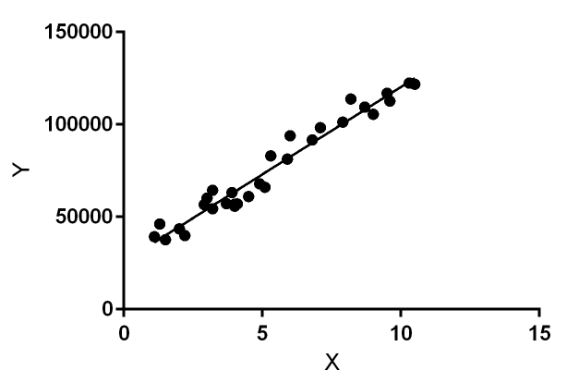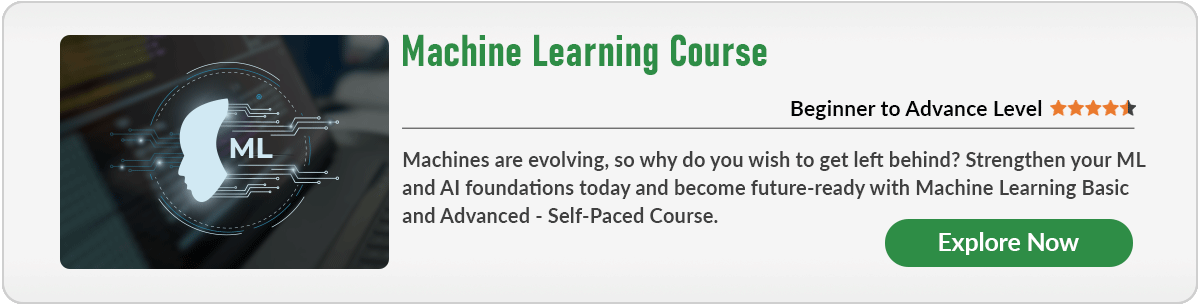Linear regression performs the task to predict a dependent variable value (y) based on a given independent variable (x). So, this regression technique finds out a linear relationship between x (input) and y(output). Hence, the name is Linear Regression.
In the figure above, X (input) is the work experience and Y (output) is the salary of a person. The regression line is the best fit line for our model.

Hypothesis function for Linear Regression :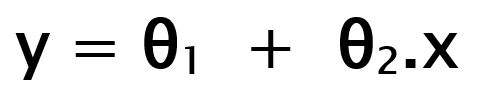While training the model we are given :
x: input training data (univariate – one input variable(parameter))
y: labels to data (supervised learning)

When training the model – it fits the best line to predict the value of y for a given value of x. The model gets the best regression fit line by finding the best θ1 and θ2 values.
θ1: intercept
θ2: coefficient of x

Once we find the best θ1 and θ2 values, we get the best fit line. So when we are finally using our model for prediction, it will predict the value of y for the input value of x.

Cost Function (J):
By achieving the best-fit regression line, the model aims to predict y value such that the error difference between predicted value and true value is minimum. So, it is very important to update the θ1 and θ2 values, to reach the best value that minimize the error between predicted y value (pred) and true y value (y).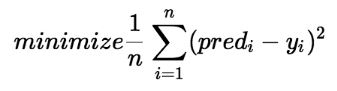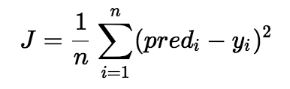Cost function(J) of Linear Regression is the Root Mean Squared Error (RMSE) between predicted y value (pred) and true y value (y).